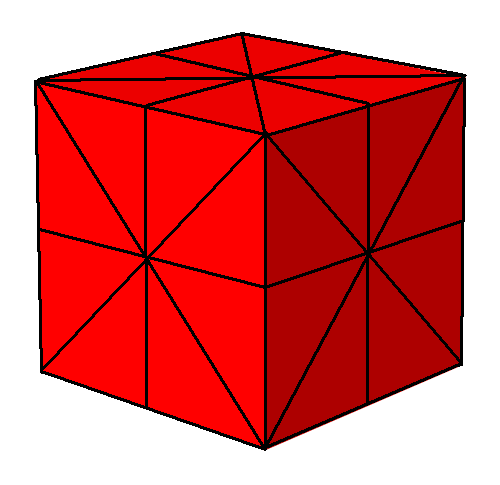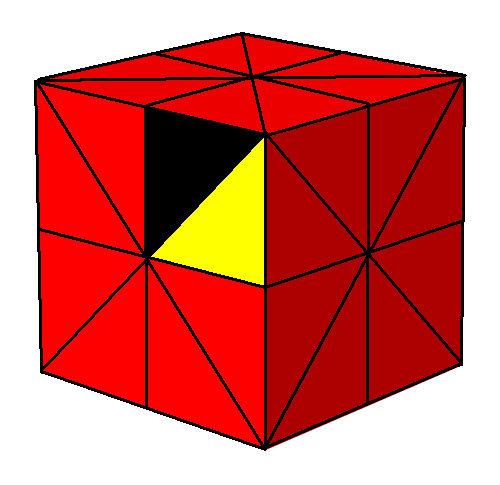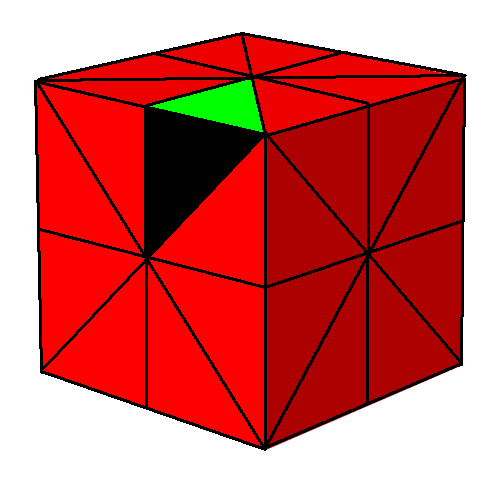## Symmetry and The Fourth Dimension (Part 3)

So, where were we? I got a bit distracted by other things. So let me review, but also push ahead a bit further. This time we’ll see what a ‘Coxeter diagram’ is. Later we’ll use Coxeter diagrams as ways to describe lots of wonderful shapes.

### Platonic solids and their Coxeter complexes

In Part 1 we started by looking at the five Platonic solids:

We saw that each Platonic solid has a bunch of symmetries where we reflect it across planes called mirrors, which all intersect at the center of the solid. If we take a sphere and slice it with these mirrors, it gets chopped up into triangles, and we get a pattern called a Coxeter complex.

Let’s pick a random Platonic solid and see how it works. For example, the dodecahedron:gives this Coxeter complex:In simple terms: we puff up our Platonic solid into a nice round sphere, and then chop its faces into triangles drawn on this sphere. That’s how it always works. And you’ll notice that each triangle has:

• one corner at the center of a face of our Platonic solid,

• one corner at the center of an edge of our Platonic solid,

• one corner at a vertex of our Platonic solid.

So any triangle determines a vertex, edge and face of our Platonic solid! But we don’t get just any old vertex, edge and face this way. The vertex has to lie on the edge, and the edge has to lie on the face.

That’s how it always works—check and see! So, let’s make up a definition:

Definition. Given a Platonic solid, a flag is a vertex, edge and face where the vertex lies on the edge and the edge lies on the face.

In fact, a Platonic solid always gives a Coxeter complex with one triangle for each flag.

### Coxeter groups

Next, in Part 2, we looked at operations that flip these triangles around. I showed you how it works for the cube. I was too lazy to puff it up into a sphere, but I chopped its surface into triangles, one for each flag:This is the lazy man’s way to draw the Coxeter complex of the cube.

Then I studied operations that flip triangles over to triangles that touch them, One operation, called V, changes which vertex of the cube our triangle contains. For example, if we start with this black triangle:the operation V changes it to this blue one:Another operation, called E, changes which edge of the cube our triangle touches:And a third, called F, changes which face of the cube our triangle lies on:Using these operations, we can get to any triangle starting from any other. But the cool part is that these operations obey some equations! For any Platonic solid, they always obey these equations:

V2 = E2 = F2 = 1

These say flipping twice the same way gets you back where you started. But there are also three equations that are more interesting. Some of these depend on which Platonic solid we’re looking at. They can be seen using pretty pictures:

• For the cube we get this relation:

(VE)4 = 1

from this picture:Get it? We flip our triangle to change which vertex of the cube it contains, then flip it to change which edge of the cube it touches… and after 8 flips we’re back to where we started, so:

VEVEVEVE = 1

or for short:

(VE)4 = 1

We get a 4 in the exponent here because each face of the cube has 4 edges and 4 vertices. So, you can easily work out how this relation goes for any Platonic solid.

• For the cube we also get this relation:

(VF)2 = 1

from this picture:We get a 2 in the exponent here because each edge of the cube contains 2 vertices and touches 2 faces. This is true no matter what Platonic solid we have!

• And finally, for the cube we get this relation:

(EF)3 = 1

from this picture:We get a 3 in the exponent here because each vertex of the cube touches 3 edges and 3 faces. So, you can easily work out how this relation goes for any Platonic solid, too.

The operations V, E, F, together with these relations, generate something called the Coxeter group of the Platonic solid. To know exactly what I mean here you need to know a wee bit of group theory, which is the study of symmetry. But even if you don’t, I hope you get the idea: the Coxeter group consists of all the ways we can flip one triangle in our Coxeter complex over to another by a sequence of V, E, and F flips.

### Coxeter diagrams

Coxeter diagrams start out being a cute way to record the equations we just saw. Later, they’ll become an amazingly system for creating and classifying highly symmetrical structures: Platonic solids in all dimensions, the solids we get by truncating these in various ways, and more.

Today I’ll draw these diagrams a bit differently than the ways you usually see in books. That’s okay: there are lots of different ways to draw them, depending on what you’re using them for.

In my current way of doing things, the Coxeter diagram of the cube looks like this:

V—4—E—3—F

There’s an edge from the letter V to the letter E, labelled by the number 4. This means

(VE)4 = 1

And there’s an edge from the letter E to the letter F, labelled by the number 3. This means

(EF)3 = 1

But there’s no edge from the V to the F. This means

(VF)2 = 1

In other words, we make up an extra rule: if an edge would be labelled by the number 2, we leave it invisible. The reason is that relations of this sort are ‘boring’: they show up a lot. For example, we’ve seen that (VF)2 = 1 for all Platonic solids.

We also don’t bother to record these relations:

V2 = E2 = F2 = 1

because these too are ‘boring’. We’ll assume relations of this sort always hold.

Now, I’ll leave you with a puzzle:

Puzzle 1. What are the Coxeter diagrams of the five Platonic solids? What patterns do you see in these?

### Coxeter diagrams of regular tilings

But so you don’t think I’m wimping out, I’ll do an example myself. The triangular tiling of the plane is not a Platonic solid:But it’s what you’d get if you tried to build a Platonic solid where 6 equilateral triangles meet at each vertex! And we can still define a Coxeter complex for it. It looks like this:There’s one triangle here for each flag in the triangular tiling. And if you stare at this picture, you can read off the equations for the Coxeter group, just as we did for the cube. We have the boring equations

V2 = E2 = F2 = 1

but also:

(VE)3 = 1

since each face of the triangular tiling has 3 vertices and 3 edges, and:

(VF)2 = 1

since each edge of this tiling contains 2 vertices and touches 2 faces, and:

(EF)6 = 1

since each vertex of the this tiling touches 6 edges and 6 faces.

So, here’s the Coxeter diagram of the triangular tiling:

V—3—E—6—F

Whoops, now I can’t resist giving you another puzzle! There are two more regular tilings of the plane, so you should try those:

Puzzle 2. What are the Coxeter diagrams of the square tiling:and the hexagonal tiling:?

### 14 Responses to Symmetry and The Fourth Dimension (Part 3)

1.Blake Stacey says:

And if you stare at this picture, you can read of the equations for the Coxeter group,

2.Michelle Beissel says:

I was able to get farther down your post and understand what I read than usual, with some things finally getting through that you have mentioned previously, so thanks for that, this post fleshed out some basic details v well. But….no dice, can’t yet apply what I know to present a possible solution to the puzzles. Some day, perhaps…

•John Baez says:

Hi! Glad it’s making more sense. If you copy how I worked out the Coxeter diagram for the cube, you can do it for the other Platonic solids. In the end, the procedure amounts to counting some things. Getting nice non-rotating pictures of these solids from Wikipedia might help. But don’t worry… we don’t all have lots of time to work on this stuff!

•Blake Stacey says:

John Baez wrote:

Getting nice non-rotating pictures of these solids from Wikipedia might help.

That might be more effective than the method I used, which was to look at the spinning polyhedra and rotate my chair.

•John Baez says:3.chrisnamastephys11uca says:

Hi John Baez,

This is an interesting post, indeed! Although I have not done group theory in a math class, but in a physical chemistry class and in a quantum mechanics class offered in a graduate program in chemical physics, I have decided to attempt the first puzzle, concerning the coexter diagrams of the five Platonic solids.

For a tetrahedron, the coexter diagram is: V-3-E-3-F.
For a cube, it is as given above: V-4-E-3-F.
For an octahedron, it is: V-3-E-3-F.
For an dodecahedron, it is: V-5-E-3-F.
And, for a icosahedron, it is: V-3-E-5-F.

I used the technique of figuring out what exponent (VE), (VF), and (EF) should be raised to, by asking for each face, edge, and vertex, respectively, how many vertices and edges, vertices and faces, and edges and faces are there respectively, with the polyhedra drawn out on paper.

The coexeter diagrams for the tetrahedron and octahedron are equivalent, whereas the dodecahedron and icosahedron have different coexter diagrams since the dodecahedron is made up of pentagonal faces, whereas the icosahedron has triangular faces, but with pentagonal cross sections across the top and bottom, symmetric about the x axis running through the center of the polyhedron.

I will try to tackle the second puzzle at some point, too.

•John Baez says:

Hi! Good work! I’m glad you enjoyed this challenge. You made one little mistake, which affects some of your conclusions. Maybe you can spot it, or someone else here can. The picture at the very top of this post is a clue.

•Blake Stacey says:

Hint: the way the spinning solids are arranged into rows is actually significant!

4.chrisnamastephys11uca says:

Hi John Baez!

Thank you for posting such interesting things on your blog. I started following your blog a little while ago (about a couple of months, I think) and really find the topics covered very fascinating–thanks for your great work!

I did find my mistake, indeed. It is for the Coxeter diagram of the octahedron, which has a Coxeter diagram of V-3-E-4-F.

All the other Coxeter diagrams for the other Platonic solids remain the same as in my previous comment, after a second look at them.

I noticed that for each of the Platonic solids, the relation$(VF)^2 = 1$

holds for each of them.

Also, the Coxeter diagrams of the cube and octahedron seem to be the ”reverse” or ”inverse” of each other with regard to the ordering between V, E, and F, whereas the dodecahedron and icosahedron Coxeter diagrams indicate that they are the “reverse” or ”inverse” of each other, which makes sense. For the cube and octahedron cases, the ”base” that unites the two pyramids of the octahedron is a square, which constitutes the base of a cube.

Hopefully, this is more complete and correct in evaluation than my previous attempt. I still have yet to figure out the second puzzle, but look forward to it!

•John Baez says:

Yes, great! The Coxeter diagram for the octahedron is

V—3—E—4—F

because it has 3 vertices and edges around each face, and 4 edges and faces around each vertex:

You write:

Also, the Coxeter diagrams of the cube and octahedron seem to be the “reverse” or “inverse” of each other with regard to the ordering between V, E, and F, whereas the dodecahedron and icosahedron Coxeter diagrams indicate that they are the “reverse” or “inverse” of each other, which makes sense.

That’s right, and that’s one of the most exciting patterns in the Platonic solids, made vividly visible by their Coxeter diagrams.

The usual term is ‘dual’: if we draw a dot in the center of each face of a Platonic solid, these dots are the vertices of another Platonic solid, its dual. The dual of the dual is the original solid (though smaller, the way I’ve described it now).

So:

• The dual of the tetrahedron is itself.

• The dual of the cube is the octahedron.

• The dual of the dodecahedron is the icosahedron.

It’ll be fun to exploit this fact in future posts, and also to see how it generalizes to 4 dimensions.

5. […] Last time I posed a puzzle: figure out the Coxeter diagrams of the Platonic solids. […]

6.chrisnamastephys11uca says:

Hi John Baez,

I saw that you posted part 4 of the Symmetry and The Fourth Dimension series and read it already and hopefully will get to it shortly. Nevertheless, I wanted to answer your second puzzle as well, for the coexter diagrams of the square tiling and hexagonal tiling.

For the square tiling, the coexter diagram is: V-4-E-4-F, whereas the coexter diagram for the hexagonal tiling is: V-6-E-3-F.

7. Remember how I defined a ‘flag’ in Part 3? […]

This site uses Akismet to reduce spam. Learn how your comment data is processed.